## Significant Figures Worksheet(Sig Figs): Definition, Examples, and Practice

Meta: Whether you’re relatively new to significant figures or are just looking for a refresher, you’ve come to the right place. Read on to learn more!

# Significant Figures (Sig Figs): Definition, Examples, and Practice

Significant figures are a vital concept when you’re working in any field that requires precision; chemistry, for example. And while it feels like a complex subject, it’s fairly simple and straightforward. In this article, we’re answering your questions about significant figures and prepping you for significant figures worksheets with lots of examples.

## Reason for Significant Figures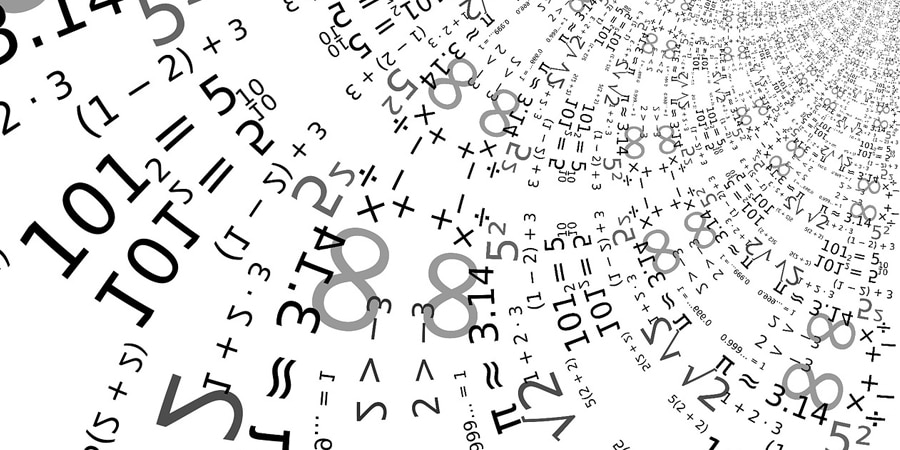We can all agree that there’s nothing worse than complicated mathematics concepts that don’t seem to have anything to do with real life. Fortunately, that’s not the case with significant figures! The primary purpose for this somewhat abstract concept is precision.

The numbers we use in everyday life, as it turns, are not that precise. When you’re working in specialized fields, however, the precision of the data is everything. Significant figures help you communicate how precisely you’ve measured and help to ensure mistakes are made with the data.

### Let’s Build a TowerHere’s an example: let’s say you’re building a tower. As you might imagine, precision matters hugely. You don’t want to get to the top of the tower only to realize your tower is taller–or shorter!–than you thought. Let’s say, then, that the steel manufacturer has measured its vertical beams to the nearest centimeter and is sending you three beams that are each 20.5 meters tall.

This measurement is precise to the centimeter, but the number doesn’t tell you anything about millimeters. This is a problem because the glass you’re cutting to cover the building in is measured to the nearest millimeter; in fact, the glass company is sending the glass to you in 15.75-meter sheets.

You know that your steel beams will stand end to end and that your finished tower will be (20.5*3) meters tall, or 61.5 meters tall. Three glass sheets will be 47.25 meters tall; too short. Four glass sheets will be 63 meters tall; too tall. You’ll need to lay three sheets of glass and cut the fourth to size. Now you need to figure out how much you should cut down that fourth sheet of glass.

### The Problem With Imprecision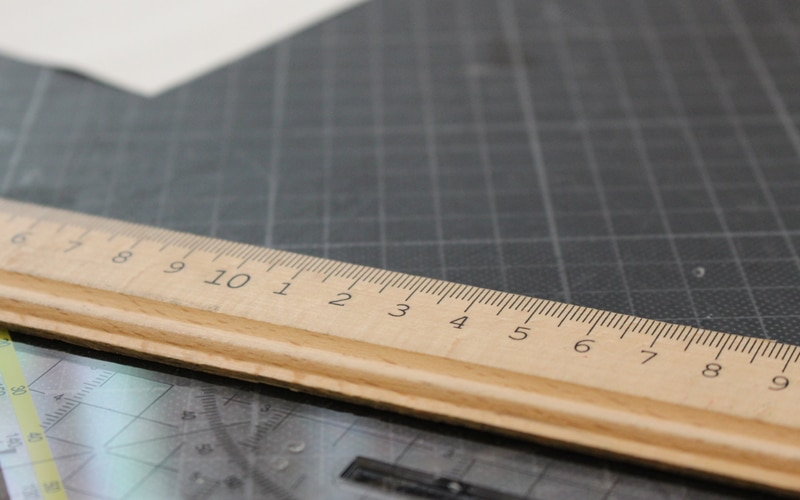Here’s the problem: your steel is measured to the centimeter, but the glass cutter cuts to the millimeter. Each steel beam is 20.5 meters, which means it’s twenty meters and five centimeters; but how many millimeters is it?

We don’t know. It could be three millimeters (20.53 meters), or it could be seven millimeters (20.57 meters). Let’s say it’s the latter, and your three steel beams each have an extra seven millimeters that you don’t know about because they’ve only been measured to the nearest centimeter.

You think you’ve only got 61.5 meters of a tower (because 20.5*3 = 61.5) but you have 61.71 meters (because 20.57*3 = 61.71). That’s a difference of .21 meters, or a whopping two centimeters and one millimeter! If you cut your glass to 61.5 meters, you’ll get to the top of the tower to install it–only to realize you’re missing two centimeters and a millimeter of glass.

Oops!

This is an oversimplified example, but it helps to illustrate how important precision is–and now you know how significant figures work, even if you don’t know the concept by that name yet. That’s for the next section!

## Rules for Significant Figures

The first thing you should remember is that significance doesn’t indicate whether a number is computed or not; it simply indicates the precision of measurement. We’ll explain; let’s start with the basic ground rules about significant figures, and then we’ll practice with some examples you might see on a significant figures worksheet:

1. If it’s not a zero, the number is a significant figure (e.g., 1, 2, 3, 4, 5, 6, 7, 8, and 9 are all significant no matter where they are in the number)
2. If a zero is between a non-zero digit, it is significant (e.g., the zeros in these numbers are significant: 507, 5007, 5000.07)
3. Trailing zeros (the zeros at the end of a number) are only significant if the number has a decimal point (e.g., 5.0500 or 5.50); they are not significant if there are no decimal points (e.g., 500 or 50) (special note: in the latter case, significance can be indicated with a bar above or below the last measured figure)
4. Leading zeros (zeros before non-zeros) are never significant (e.g., 005 or 0.005)

### EXAMPLES

• 51 has two significant figures (5 and 1; all numbers are non-zeros)
• 1234.56 has six significant figures (1, 2, 3, 4, 5 and 6; all numbers are non-zeros)
• 505.5505 has seven significant figures (5, 5, 0, 5, 5, 0, and 5; all non-zeros are significant, and all zeros between non-zeros are significant)
• 0.0057 has two significant figures (5 and 7; the zeros are leading and are not significant)
• 500 and 50 each have one significant figure (5 and 5; trailing zeros are not significant unless there is a decimal point)
• 5.0500 has five significant figures (5, 0, 5, 0, and 0; the first zero is between two non-zeros and the trailing zeros are significant because the number contains a decimal)
• 0.00050500 has five significant figures also (remember that leading zeros do not count)
• 1300 has two significant figures (one and three) while 1300. has four significant figures (1, 3, 0, and 0) because the latter number has a decimal point

## Multiplying, Dividing, Adding, and Subtracting with Significant Figures

Before we get into the mechanics of multiplying, dividing, adding, and subtracting with significant figures, you’ll first need to know how to round up significant figures. For example, if you have a number (let’s say 54.896) that has five significant figures, and you need to round it up to three significant figures, how do you do that?

### Rounding Up

Here’s how:

1. Identify the significant figures (in our example, there are five significant numbers: 5, 4, 8, 9, and 6)
2. Identify the last of the desired significant figures (we know we need three significant figures, so eight, the third significant figure from the left, is the last significant figure)
3. Look at the number to the right of the significant figure. If it is five or greater, add one to the last significant figure (in our example, eight is followed by nine; nine is greater than 5; therefore, eight is rounded up by 1 to 9 so that our new number is 54.9). If, however, the number is four or less, simply remove all the remaining numbers to the left (don’t replace them with zeros)

Here’s another example using 0.01234500:

If you want to round it to three significant figures, the answer would be 0.0123 (leading zeros are not significant; 4 is less than five, so 4 and all subsequent numbers are removed).

Special note: rounding significant figures is different than rounding decimals. For example, if you wanted to round our example number to three decimal places, the answer would be .012, which is a different number altogether from a precision standpoint.

### Multiplying and Dividing

When you begin working seriously with multiplying and dividing, you’ll notice how quickly things can move from precise to imprecise. For example, let’s go back to our tower example and say we want to measure the area of our glass sheets. They are 15.75 meters tall and 2.5 meters wide.

A simple calculation (15.75*2.5=39.375) tells us the area is 39.375, but if you’re thinking, “wait! That measurement is more precise than what we measured!” you’d be right. To keep our quotients and products as precise as our initial measurements, we need to round up (or down).

The general rule is that the significant figures in the answer cannot be more than the smallest amount of significant figures used in the equation. In our glass sheets example, the two numbers we multiplied are 15.75 (which has four significant figures) and 2.5 (which has two significant figures). The smallest number of significant figures in either of these factors is two, so our answer can’t have more than two significant figures.

Our answer (39.375) has five significant figures, with 9 being the second significant figure. The number to the right of 9 is 3, which is less than 5, so we simply discard 3 and everything to its right. Our answer, then, is 40. (9 rolls to the 0 and the 3 becomes a 4; also note the use of the decimal!).

Adding and subtracting numbers with different amounts of significant digits is very similar to multiplying and dividing, except that the rounding up (or down) happens before the calculation.

Back to our tower example, let’s say you had one steel beam that was 20.5 meters and one that was 20.45 meters, you are dealing with two different levels of precision. The first beam has three significant figures and is less precise than the second beam, which has four significant figures.

If you add them together as is, it’s just messy. So, you need to round up the more precise number so that it matches the least precise number. That is, you would round up 20.45 to 20.5 and add both numbers (20.5 + 20.5 = 41.0; note that the answer is also precise to three significant figures).

Hopefully, significant figures are no longer mystifying, and you’re ready to tackle your significant figures worksheet; good luck!

## Dimensional Analysis: Definition, Examples, And Practice

If you’ve heard the term “dimensional analysis,” you might find it a bit overwhelming. While there’s a lot to “unpack” when learning about dimensional analysis, it’s a lot easier than you might think. Learn more about the basics and a few examples of how to utilize the unique method of conversion.

# Dimensional Analysis: Definition, Examples, and PracticeAs a student of Biology or any of the sciences, you will have to use math of some kind, and there’s a good chance that you will find dimensional analysis (or unit analysis) to be helpful. Math equations and other conversions can be overwhelming for some, but dimensional analysis doesn’t have to be; once you learn it, it’s relatively easy to use and understand.

We’ll give you the basics and give you some easy-to-understand examples that you might find on a dimensional analysis worksheet so that you can have a general understanding about what it is and how to use the technique in all types of applications as you continue to take science courses.

## What Is Dimensional Analysis?As we mentioned, you may hear dimensional analysis referred to as unit analysis; it is often also known as factor-label method or the unit factor method. A formal definition of dimensional analysis refers to a method of analysis “in which physical quantities are expressed in terms of their fundamental dimensions that is often used.”

Most people might agree that this definition needs to be broken down a bit and simplified. It might be easier to understand this method of analysis if we look at it as a method of solving problems by looking converting one thing to another.

While dimensional analysis may seem like just another equation, one of the unique (and important) parts of the equation is that the unit of measurement always plays a role in the equation (not just the numbers).

We use conversions in everyday life (such as when following a recipe) and in math class or in a biology course. When we think about dimensional analysis, we’re looking at units of measurement, and this could be anything from miles per gallon or pieces of pie per person.

Many people may “freeze up” when they see a dimensional analysis worksheet or hear about it in class, but if you’re struggling with some of the concepts, just remember that it’s about units of measurements and conversion. Dimensional analysis is used in a variety of applications and is frequently used by chemists and other scientists.

## The Conversion Factor in Dimensional AnalysisOne important thing to consider when using dimensional analysis is the conversion factor. A conversion factor, which is always equal to 1, is a fraction or numerical ratio that can help you express the measurement from one unit to the next.

When using a conversion factor, the values must represent the same quantity. For example, one yard is the same as three feet or seven days is the same as one week. Let’s do a quick example of a conversion factor.

Imagine you have 20 ink pens and you multiply that by 1; you still have the same amount of pens. You might want to find out how many packages of pens that 20 pens equal and to figure this out, you need your conversion factor.Now, imagine that you found the packaging for a set of ink pens and the label says that there are 10 pens to each package. Your conversion factor ends up being your conversion factor. The equation might look something like this:

20 ink pens x 1 package of pens/10 pens = 2 packages of ink pens. We’ve canceled out the pens (as a unit) and ended up with the package of pens.

While this is a basic scenario, and you probably wouldn’t need to use a conversion factor to figure out how many pens you have, it gives you an idea of what it does and how it works. As you can see, conversion factors work a lot like fractions (working with numerators and denominators)

Even though you’re more likely to work with more complex units of measurement while in chemistry, physics, or other science and math courses, you should have a better understanding of using the conversion factor in relation to the units of measurement.

## Steps For Working Through A Problem Using Dimensional AnalysisLike many things, practice makes perfect and dimensional analysis is no exception. Before you tackle a dimensional analysis that your instructor hands to you, here are some tips to consider before you get started.

• Write down your problem in a way that you can understand
• Consider a simple math equation and don’t forget the conversion factors
• Remember, some of the units should cancel out, resulting in the unit you want
• Double-check and retry if you have to
• The answer you come up with should make sense to you

To help you understand the basic steps we are using an easy problem that you could probably figure out fairly quickly. The question is: How many seconds are in a day?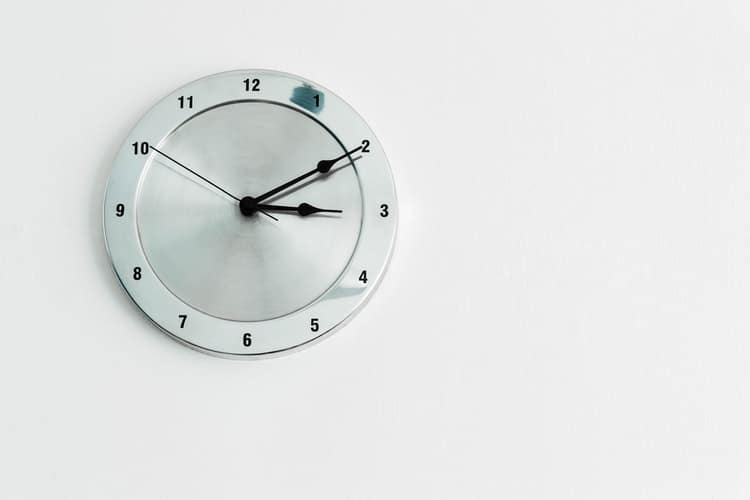First, you need to read the question and determine the unit you want to end up with; in this case, you want to figure out “seconds in a day.” To turn this word problem into a math equation, you might decide to put seconds/day or sec/day.

The next step is to figure out what you already know. You know that there are 60 seconds to one minute and you also know that there are 24 hours in one day; all of these units work together, and you should be able to come up with your final unit of measurement. Again, it’s best to write down everything you know into an equation.

After you’ve done a little math, your starting factor might end up being 60 seconds/1 minute. Next, you will need to work your way into figuring out how many seconds per hour. This equation will be 60 seconds/1 minute x 60 minutes/1 hour. The minutes cancel themselves out, and you have seconds per hour.

Remember, you want to find out seconds per day so you’ll need to add another factor that will cancel out the hours. The equation should be 60 seconds/1 minute x 60 minutes/1 hour x 24 hours/1 day. All units but seconds per day should cancel out and if you’ve done your math correctly 86,400 seconds/1 day.

When doing a dimensional analysis problem, it’s more important to pay attention to the units and make sure you are canceling out the right ones to get the final product. Doing your math correctly important, but it’s easier to double-check than trying to backtrack and figure out how you ended up with the wrong unit.

Our example is relatively simple, and you probably had no problem getting the right answer or using the right units. As you work through your science courses, you will be faced with more difficult units to understand. While dimensional analysis will undoubtedly be more challenging, just keep your eye on the units, and you should be able to get through a problem just fine.

## Why Use Dimensional Analysis?As we’ve demonstrated, dimensional analysis can help you figure out problems that you may encounter in your everyday. While you’re likely to explore dimensional analysis a bit more as you take science courses, it can be particularly helpful for Biology students to learn more.

Some believe that dimensional analysis can help students in Biology have a “better feel for numbers” and help them transition more easily into courses like Organic Chemistry or even Physics (if you haven’t taken those courses yet).

Can you figure out a math equation or a word problem without dimensional analysis? Of course, and many people have their own ways of working through a problem. If you do it correctly, dimensional analysis can actually help you answer a problem more efficiently and accurately.

If you want to practice dimensional analysis, there are dozens of online dimensional analysis worksheets. While many of them are pretty basic or geared towards specific fields of study like Chemistry, we found a worksheet that has an interesting variety. Test out what we’ve talked about and check your answers when you’re done.

• How many minutes are in 1 year?
• Traveling at 65 miles/hour, how many minutes will it take to drive 125 miles to San Diego?
• Convert 4.65 km to meters
• Convert 9,474 mm to centimeters
• Traveling at 65 miles/hour, how many feet can you travel in 22 minutes? (1 mile = 5280 feet)

## Volume of Irregular Object Sample Lab 1

 Volume of an Irregular ObjectIntroduction

Everything is made of matter, and matter has both chemical and physical properties. Physical properties of matter are observable and include mass, volume, and density. Mass is the amount of matter in an object and can be found by using an electronic balance or scale. Volume is the amount of space an object occupies and can be determined by measuring the amount of water that a submerged object displaces. The formula for determining density is:

Density = Mass of the object / Volume of the object

Hypothesis

Determining the volume of an irregularly shaped object can help determine its density.

Materials

The materials used in this lab included a rubber stopper, small rock, shell, 100 ml graduated cylinder, electronic balance, water, paper, and pencil.

Methods

Obtain three, irregularly shaped objects — a rubber stopper, a shell, and a rock. Estimate the volume and mass of each object and record this in the data table. Use an electronic balance to mass each object and record the masses in the data table. Fill a graduated cylinder approximately half way with water and record this initial volume. Place one of the objects into the graduated cylinder and record the final volume. Subtract the initial volume of the water from the final volume of water to find the volume of the water the object displaced. Record this as the object’s volume in the data table. Remove the first object and repeat this process of determining volume for the other two objects. Determine each object’s density by dividing their mass by their volume. Record each density in the data table.

Results

 Data Table Object estimated mass (g) estimated volume (ml) actual mass (g) initial volume of water (ml) final volume of water (ml) volume of object (ml) density (g/ml) Rubber stopper 19.3 10 9.3 50 56 6 1.55 Shell 6.0 2 3.3 50 51.5 1.5 2.2 Rock 10.0 5 9.3 50 55 5 1.86

Discussion and Conclusion

Objects that are irregular in shape can have their volume determined by measuring the amount of water that they displaced when submerged in a known amount of water. The increase in volume from the initial water level to the final water level is the volume of the object. Once the volume is determined and the actual mass measured then the object’s density can also be determined. The formula for finding density is the mass of the object divided by the volume of the object. For example, since the rock had a mass of 9.3 grams and a volume of 5 ml, the density of the rock was determined to be 1.86 grams/ millimeters.BACK

## Water Properties Notes

 Water Properties States of Water Adhesion and Cohesion Surface Tension Capillary ActionThe States of WaterWater has three states. Below freezing water is a solid (ice or snowflakes), between freezing and boiling water is a liquid, and above its boiling point water is a gas. There are words scientists use to describe water changing from one state to another. Water changing from solid to liquid is said to be melting. When it changes from liquid to gas it is evaporating. Water changing from gas to liquid is called condensation (An example is the ‘dew’ that forms on the outside of a glass of cold soda). Frost formation is when water changes from gas directly to solid form. When water changes directly from solid to gas the process is called sublimation.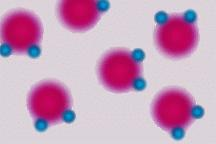Gas Liquid Solid

Most liquids contract (get smaller) when they get colder. Water is different. Water contracts until it reaches 4 C then it expands until it is solid. Solid water is less dense that liquid water because of this. If water worked like other liquids, then there would be no such thing as an ice berg, the ice in your soft drink would sink to the bottom of the glass, and ponds would freeze from the bottom up!

Water is found on Earth in all three forms. This is because Earth is a very special planet with just the right range of temperatures and air pressures.Water is attracted to other water. This is called cohesion. Water can also be attracted to other materials. This is called adhesion.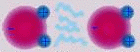The oxygen end of water has a negative charge and the hydrogen end has a positive charge. The hydrogens of one water molecule are attracted to the oxygen from other water molecules. This attractive force is what gives water its cohesive and adhesive properties.Surface Tension

Surface tension is the name we give to the cohesion of water molecules at the surface of a body of water. Try this at home: place a drop of water onto a piece of wax paper. Look closely at the drop. What shape is it? Why do you think it is this shape?

What is happening? Water is not attracted to wax paper (there is no adhesion between the drop and the wax paper). Each molecule in the water drop is attracted to the other water molecules in the drop. This causes the water to pull itself into a shape with the smallest amount of surface area, a bead (sphere). All the water molecules on the surface of the bead are ‘holding’ each other together or creating surface tension.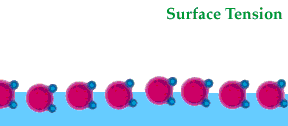Surface tension allows water striders to ‘skate’ across the top of a pond. You can experiment with surface tension. Try floating a pin or a paperclip on the top if a glass of water. A metal pin or paper clip is heavier than water, but because of the surface tension the water is able to hold up the metal.

Surface tension is not the force that keeps boats floating.Capillary Action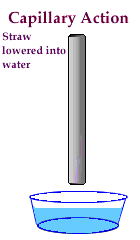Surface tension is related to the cohesive properties of water. Capillary action however, is related to the adhesive properties of water. You can see capillary action ‘in action’ by placing a straw into a glass of water. The water ‘climbs’ up the straw. What is happening is that the water molecules are attracted to the straw molecules. When one water molecule moves closer to a the straw molecules the other water molecules (which are cohesively attracted to that water molecule) also move up into the straw. Capillary action is limited by gravity and the size of the straw. The thinner the straw or tube the higher up capillary action will pull the water (Can you make up an experiment to test this?).

Plants take advantage of capillary action to pull water from the into themselves. From the roots water is drawn through the plant by another force, transpiration.

## Why Boats Float

How does a heavy boat float?

 A boat, or any other object designed to float, is based on a theory by a very old guy, even older than Capt. Matt. Though he is old and, by the way, dead, he was really a cool guy and his name was Archimedes (Ark-i’-meed-eez). His principle, cleverly named the Archimedes’ Principle, explains how things float.If you fill your bathtub with water, what happens when you get in? The water rises, right? (And sometimes goes over the side.) That is because you “displaced” some of the water with your body and it had to go somewhere. The key to floating is that the object must displace an amount of water which is equal to its own weight.For example, suppose you had a block of wood that was 1 foot square. Let’s say that this block of wood weighs about 50 pounds. Now say we lower that wood into the water. The wood will move down into the water until it has displaced 50 pounds of water. That means that fifty pounds of water are pushing back up on the block and making it float.The principle of floating is pretty easy, however, if you want to remain inside the boat and actually get where you want to go, your boat must have “stability” as well as being able to float. Stability means that it is designed not to tip over easily. That doesn’t mean it won’t ever tip over.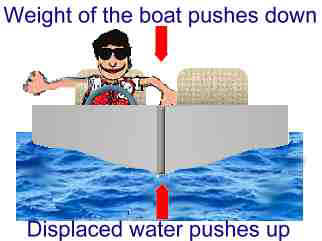On a large ship like an ocean liner or tanker, the movement of one person doesn’t affect the stability of the ship because it was designed to safely carry lots of weight. But on a small boat, like a fishing boat, your weight and the weight of your gear (and where you put it) has an effect on the stability of the boat.A boat is said to “heel” (no not the one on your foot) when it leans over to one side. This is why you never want to sit or step onto the side of a boat. Your weight could make it “heel” too much and it may tip over. You should also balance the weight of all the stuff you bring with you. In a small boat, you and your gear should always stay low and to the center of the boat. When getting into a small boat, always try to step into the center and keep “one hand for yourself and one for the boat.”Of course, because you have on your PFD and are displacing enough water to float, you would be okay, just a little wet and cold. If this should ever happen to you and you can’t right the boat (turn it back over), stay with the boat, blow your whistle or yell for help.So . . . the next time someone says “Whatever floats your boat” tell them about Archimedes and stability and why it’s a very good idea to always wear your life jacket!

## Electricity Elementary

 ELECTRICITYStandards:PS.7.4.2   Classify electrical conductors and electrical insulatorsPS.7.4.3   Construct simple circuits from circuit diagramsNotes: What is Electricity? – Just the basics about electricityCircuits Can Be Electrifying! – All types of circuits covered — open, closed, series, and parallelStatic Electricity and Dryer Sheets! – Covers that problem of static cling in your clothesElectricity and Magnetism –  Tells students how these two are relatedConductors and Insulators – What will and what won’t conduct an electric current and whyInteractive Activities: Let’s Go Swimming with Electric Eels! —Great interactive on insulators Electric Current and Voltage — Another great BBC interactive! Build an Online Circuit for Annie’s Home! — Good way for students to build and explore working circuits in their homes Circuits and Conductors — Test different substances by adding them into the circuit and seeing if the bulb will light Using Electricity! — Complete circuits and then take a quiz on electricity to see what you’ve learnedSmart Board Activities:Labs:Complete the Circuit Game! — Elementary students will love this activity by competing with each other for the most steady hand!  They’ll learn something about open and closed circuits too!!Great Links: The Energy Story – This site has great kid-friendly interactives on all aspects of energy! Where Does Our Town’s Electricity Come From? — This is great for those GT kids that want to know more about electricity.  Students will visit and electrical substation like one in their town. Learn About Electricity Online — Students learn what causes electricity, how to build circuits, conducting and insulating materials, and much more! Electricity Web Quest – This site contains several links on topics such as static electricity, circuits and safety.

## BI Sample 2 Lab Volume of Object

 Volume of an Irregular Object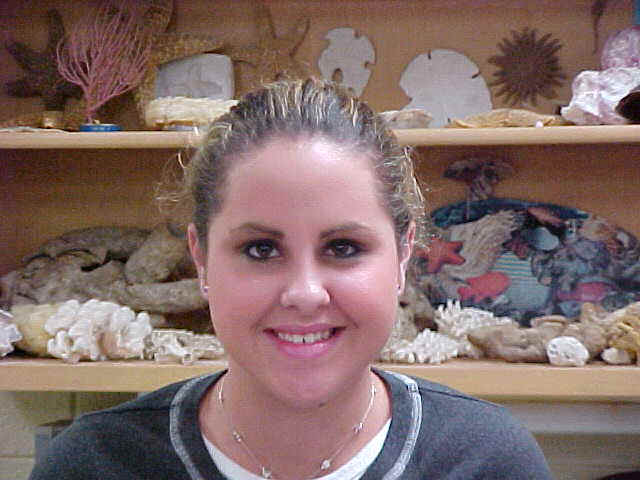Introduction

Everything is made of matter. Matter has physical and chemical properties. Physical properties are observable, such as mass, volume, and density. Mass is a fundamental property of an object generally regarded as equivalent to the amount of matter in the object. Volume is the amount of space it takes up. Density is the thickness of it the formula for density is D=M/V. The purpose of this experiment was to find the mass, volume, and density of three different objects.

Hypothesis

Determining the volume of an irregularly shaped object can help in determining density.

Materials

The materials used included a rubber stopper, a rock, a shell, a 100mL graduated cylinder, water, electronic balance, paper, and pencil.

Methods:

Obtain rubber a stopper, a shell, and a rock. Estimate and record the mass and volume of the three objects. Weigh and record each object. Take the graduated cylinder and fill halfway with water and record the volume. Add one object and record the new volume. Subtract initial volume from final, and record. Repeat with the other three objects.

Results:

 Object Estimated mass (g) Estimated volume (ml) Actual mass (g) Volume of H2O (ml) Volume of object + H2O (ml) Object’s Volume (ml) Density D=m/v(g/ml) Rubber Stopper 8g 65ml 8.3g 50ml 56ml 6ml 1.38g/ml Shell 2g 55ml 3.1g 50ml 53ml 3ml 1g/ml Rock 4g 60ml 7.8g 50ml 54ml 4ml 1.95g/ml

1. How did you determine the object’s:

a. Mass? Weighed it on a electronic balance

b. Volume? Put it in the water and measured the volume then subtracted the volume of the water before the object.

c. Density? Divided mass into volume

2. How did your estimates of mass and volume compare to the actual mass and volume of each object?

Our estimates of mass were close to the actual mass. Our estimates of volume were off not close to the actual volume at all.

3. Objects will sink if they are denser than water. Explain why ships made of steel float instead of sinking since steel is denser than water.

Because the ship has a hallow cavity with trapped air in it.

Error Analysis:

The volume of the water might not have been measured correctly.

Discussion and Conclusion:

The volume of the rubber stopper, rock and shell were determined by submerging them in a graduating cylinder containing water. The original water level in the cylinder was subtracted from the final water level to get the volume (ml) of each object. The actual mass (g) of each object was obtained by placing each on an electronic balance. Density could then be determined by dividing the mass of each object by its volume. The data showed the rock to have the greatest density at 1.95 g/ml with the rubber stopper 1.38 g/ml and the shell 1 g/ml being less dense.